Courses

# Fourier Transform - Mathematical Methods of Physics, UGC - NET Physics Physics Notes | EduRev

## Physics : Fourier Transform - Mathematical Methods of Physics, UGC - NET Physics Physics Notes | EduRev

The document Fourier Transform - Mathematical Methods of Physics, UGC - NET Physics Physics Notes | EduRev is a part of the Physics Course Physics for IIT JAM, UGC - NET, CSIR NET.
All you need of Physics at this link: Physics

The Fourier transform is a generalization of the complex Fourier series in the limit as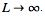. Replace the discreteAn with the continuous  F(k) dk while letting n/L → k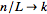. Then change the sum to an integral, and the equations become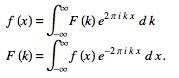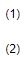Here,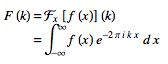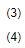is called the forward (-i) Fourier transform, and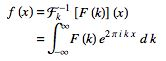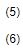is called the inverse (+i) Fourier transform. The notation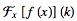is introduced in Trott (2004, p. xxxiv), and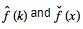are sometimes also used to denote the Fourier transform and inverse Fourier transform, respectively (Krantz 1999, p. 202).

Note that some authors (especially physicists) prefer to write the transform in terms of angular frequency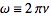instead of the oscillation frequency v. However, this destroys the symmetry, resulting in the transform pair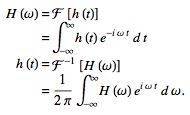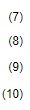To restore the symmetry of the transforms, the convention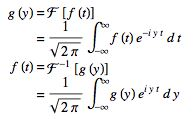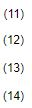sometimes used (Mathews and Walker 1970, p. 102).

In general, the Fourier transform pair may be defined using two arbitrary constants a and b as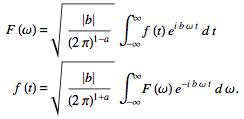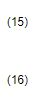The Fourier transform F(k) of a function f(x) is implemented the Wolfram Language as FourierTransform[f, x, k], and different choices of a and b can be used by passing the optional FourierParameters-> {a, b} option. By default, the Wolfram Language takes FourierParameters as (0,1). Unfortunately, a number of other conventions are in widespread use. For example, (0,1) is used in modern physics, (1,-1) is used in pure mathematics and systems engineering, (1,1) is used in probability theory for the computation of the characteristic function, (-1,1) is used in classical physics, and (0, -2π) is used in signal processing. In this work, following Bracewell (1999, pp. 6-7), it is always assumed that a = 0 and b = -2π unless otherwise stated. This choice often results in greatly simplified transforms of common functions such as 1,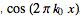, etc.

Since any function can be split up into even and odd portions E(x) and O(x),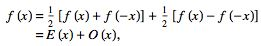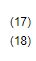a Fourier transform can always be expressed in terms of the Fourier cosine transform and Fourier sine transform as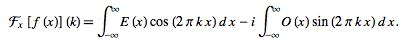(19)

A function f(x) has a forward and inverse Fourier transform such that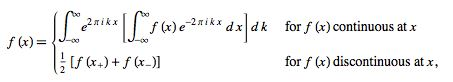(20)

provided that

1..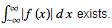2. There are a finite number of discontinuities.

3. The function has bounded variation. A sufficient weaker condition is fulfillment of the Lipschitz condition

(Ramirez 1985, p. 29). The smoother a function (i.e., the larger the number of continuous derivatives), the more compact its Fourier transform.

The Fourier transform is linear, since if f(x) and g(x) have Fourier transforms F(k) and G(k), then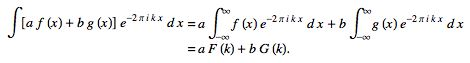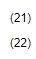Therefore,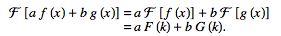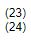The Fourier transform is also symmetric since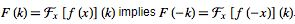.

Let f * g denote the convolution, then the transforms of convolutions of functions have particularly nice transforms,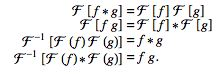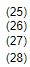The first of these is derived as follows: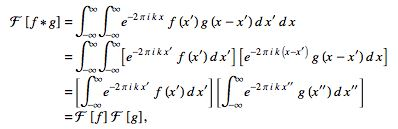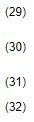where  .x" ≡ x - x'

There is also a somewhat surprising and extremely important relationship between the autocorrelation and the Fourier transform known as the Wiener-Khinchin theorem. Let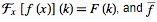denote the complex conjugate of f, then the Fourier transform of the absolute square of F(k) is given by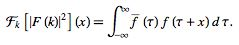(33)

The Fourier transform of a derivative f'(x) of a function f(x) is simply related to the transform of the function f(x)  itself. Consider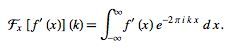(34)

Now use integration by parts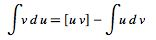(35)

with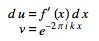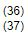and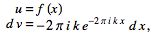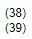then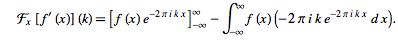(40)

The first term consists of an oscillating function times f(x). But if the function is bounded so that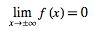(41)

(as any physically significant signal must be), then the term vanishes, leaving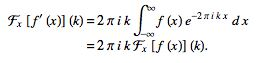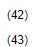This process can be iterated for the nth derivative to yield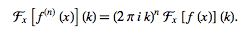(44)

The important modulation theorem of Fourier transforms allows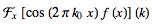to be expressed in terms of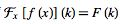as follows,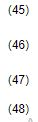Since the derivative of the Fourier transform is given by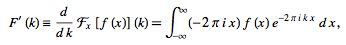(49)

it follows that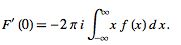(50)

Iterating gives the general formula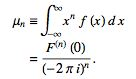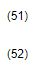The variance of a Fourier transform is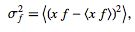(53)

and it is true that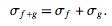(54)

If f(x) has the Fourier transform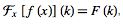, then the Fourier transform has the shift property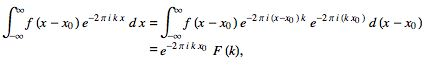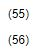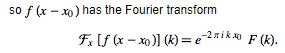(57)

If f(x) has a Fourier transform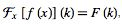, then the Fourier transform obeys a similarity theorem.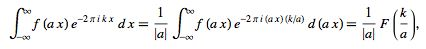(58)

so f(a x) has the Fourier transform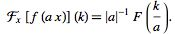(59)

The "equivalent width" of a Fourier transform is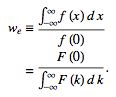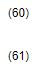The "autocorrelation width" is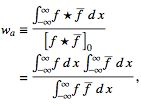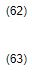where f * g denotes the cross-correlation of f andand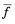is the complex conjugate.

Any operation on f(x) which leaves its area unchanged leaves F(0) unchanged, since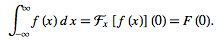(64)

The following table summarized some common Fourier transform pairs.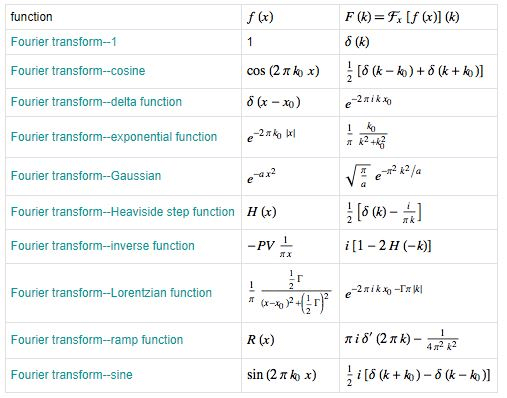In two dimensions, the Fourier transform becomes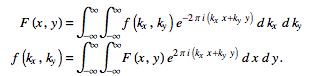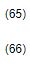Similarly, the n-dimensional Fourier transform can be defined for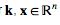by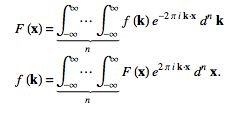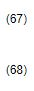159 docs

,

,

,

,

,

,

,

,

,

,

,

,

,

,

,

,

,

,

,

,

,

,

,

,

;